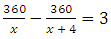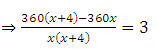##### Get a free home demo of LearnNext

Available for CBSE, ICSE and State Board syllabus.
Call our LearnNext Expert on 1800 419 1234 (tollfree)
OR submit details below for a call back

clear

karan Sharma

Nov 1, 2013

# A person on tour has Rs 360 for his expenses.

A person on tour has Rs 360 for his expenses. If he extends his tour for 4 days, he has to cut down his daily expenses by Rs 3. Find the original duration of the tour.

Kishore Kumar

Amount with the person = Rs 360 Let the original number of duration of the tour be x days Daily expenses per day = Rs 360/x Given that his tour is extended for 4 days Hence daily expenses per days = Rs 360/(x + 4) Therefore⇒ 360x + 1440 – 360x = 3(x2 + 4x) ⇒  1440 = 3x2 + 12x ⇒  3x2 + 12x – 1440 = 0 ⇒  x2 + 4x – 480 = 0 ⇒  x2 + 24x – 20x – 480 = 0 ⇒  x(x + 24) – 20(x + 24) = 0 ⇒  (x + 24)(x – 20) = 0 ⇒  (x + 24) = 0 or (x – 20) = 0 ∴ x = –24 or x = 20 Since x cannot be negative, x = 20 Hence his original duration of the tour is Rs 20

Syeda

Answer. Amount with the person = Rs 360 Let the original number of duration of the tour be x days Daily expenses per day = Rs 360/x Given that his tour is extended for 4 days Hence daily expenses per days = Rs 360/(x + 4) Therefore⇒ 360x + 1440 – 360x = 3(x2 + 4x) ⇒  1440 = 3x2 + 12x ⇒  3x2 + 12x – 1440 = 0 ⇒  x2 + 4x – 480 = 0 ⇒  x2 + 24x – 20x – 480 = 0 ⇒  x(x + 24) – 20(x + 24) = 0 ⇒  (x + 24)(x – 20) = 0 ⇒  (x + 24) = 0 or (x – 20) = 0 ∴ x = –24 or x = 20 Since x cannot be negative, x = 20 Hence his original duration of the tour is Rs 20SME Approved
###### Like NextGurukul? Also explore our advanced self-learning solution LearnNext
Offered for classes 6-12, LearnNext is a popular self-learning solution for students who strive for excellence
Explore
Animated Video
lessons
All India
Test Series
Interactive Video
Experiments
Best-in class
books